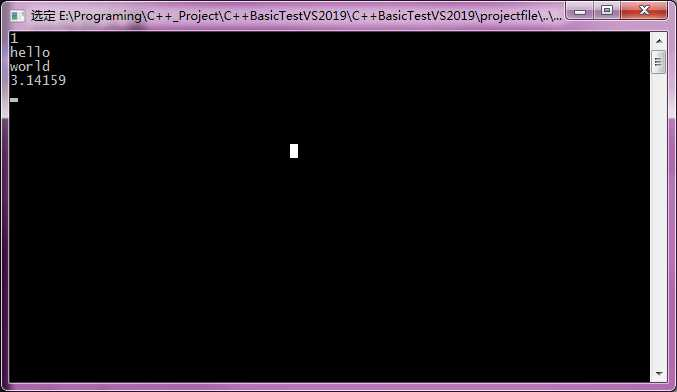# 1 C++11解析并获取可变参数列表中的所有参数

#include <iostream>
#include <vector>
#include <string>
#include <algorithm>

class ArgBase
{
public:
ArgBase() {}
virtual ~ArgBase() {}
virtual void PrintArgs() = 0;
};

template <typename T>
class Arg : public ArgBase
{
public:
Arg(T arg) : m_Arg(arg) {}
virtual ~Arg() {}
virtual void PrintArgs()
{
std::cout << m_Arg << std::endl;
}
public:
T m_Arg;
};

class ArgArray : public std::vector<ArgBase*>
{
public:
ArgArray() {}
~ArgArray()
{
std::for_each(begin(), end(), [](ArgBase* p) { delete p; });
}
};

template <typename T>
static void ParseArgs(ArgArray& argArray, T t)
{
argArray.push_back(new Arg<T>(t));
}

template<typename T, typename ...Args>
static void ParseArgs(ArgArray& argArray, T t, Args&& ...args)
{
ParseArgs(argArray, t);
ParseArgs(argArray, args...);
}

template<typename ...Args>
void ParseTest(Args&&... args)
{
ArgArray argArray;
ParseArgs(argArray, args...);

// 打印可变参数列表中的参数
for (int i = 0; i < argArray.size(); ++i)
{
argArray[i]->PrintArgs();
}

}

int main()
{
ParseTest(1, "hello", "world", 3.1415926);

getchar();

return 0;
}